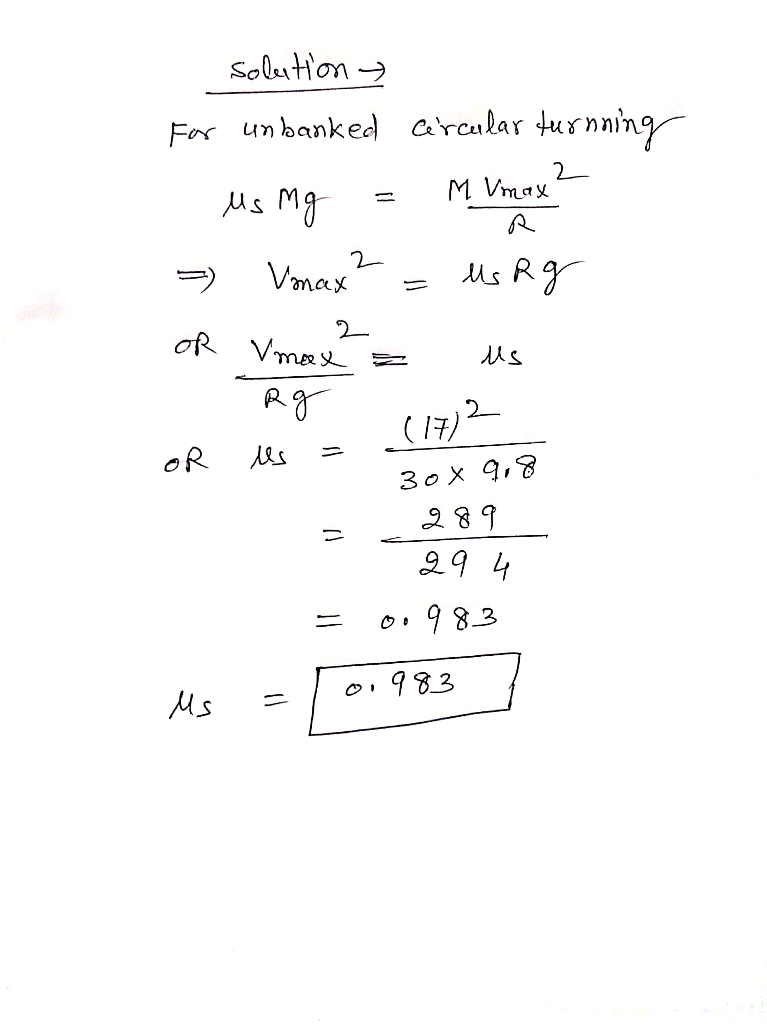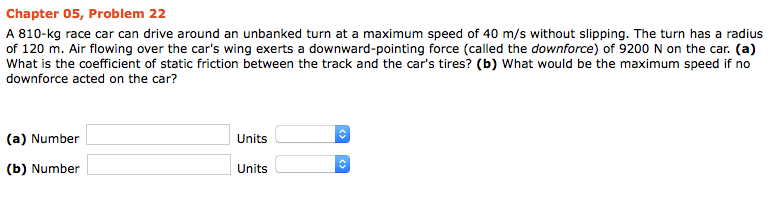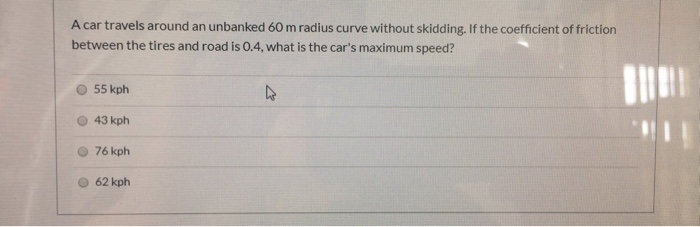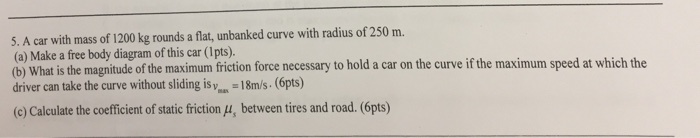Question

# A particular unbanked turn in the road is shaped like a circle with a radius of...

A particular unbanked turn in the road is shaped like a circle with a radius of 30 meters. A car
with a mass of 1500 kg can safely go around this turn at a maximum speed of 17 m/s. What is the
coefficient of static friction between the car's tires and the road?#### Earn Coins

Coins can be redeemed for fabulous gifts.

Similar Homework Help Questions
• ### A 960-kg race car can drive around an unbanked turn at a maximum speed of 45...

A 960-kg race car can drive around an unbanked turn at a maximum speed of 45 m/s without slipping. The turn has a radius of 160 m. Air flowing over the car's wing exerts a downward-pointing force (called the downforce) of 13000 N on the car. (a) What is the coefficient of static friction between the track and the car's tires? (b) What would be the maximum speed if no downforce acted on the car?

• ### A 810-kg race car can drive around an unbanked turn at a maximum speed of 40...A 810-kg race car can drive around an unbanked turn at a maximum speed of 40 m/s without slipping. The turn has a radius of 120 m. Air flowing over the car's wing exerts a downward-pointing force (called the downforce) of 9200 N on the car. What is the coefficient of static friction between the track and the car's tires? What would be the maximum speed if no downforce acted on the car?

• ### A 860-kg race car can drive around an unbanked turn at a maximum speed of 44...

A 860-kg race car can drive around an unbanked turn at a maximum speed of 44 m/s without slipping. The turn has a radius of 140 m. Air flowing over the car's wing exerts a downward-pointing force (called the downforce) of 11000 N on the car. (a) What is the coefficient of static friction between the track and the car's tires? (b) What would be the maximum speed if no downforce acted on the car?

• ### A 900-kg race car can drive around an unbanked turn at a maximum speed of 42...

A 900-kg race car can drive around an unbanked turn at a maximum speed of 42 m/s without slipping. The turn has a radius of 170 m. Air flowing over the car's wing exerts a downward-pointing force (called the downforce) of 10000 N on the car. (a) What is the coefficient of static friction between the track and the car's tires? (b) What would be the maximum speed if no downforce acted on the car?

• ### A car is safely negotiating an unbanked circular turn at a speed of 18 m/s. The road is dry, and the maximum static fri...

A car is safely negotiating an unbanked circular turn at a speed of 18 m/s. The road is dry, and the maximum static frictional force acts on the tires. Suddenly a long wet patch in the road decreases the maximum static frictional force to one third of its dry-road value. If the car is to continue safely around the curve, to what speed must the dirver slow the car?

• ### A car travels around an unbanked 60 m radius curve without skidding, If the coefficient of...A car travels around an unbanked 60 m radius curve without skidding, If the coefficient of friction between the tires and road is 0.4, what is the car's maximum speed? 55 kph 47 43 kph 76 kph 62 kph

• ### A car is safely negotiating an unbanked circular turn at a speed of 29 m/s. The...

A car is safely negotiating an unbanked circular turn at a speed of 29 m/s. The road is dry, and the maximum static frictional force acts on the tires. Suddenly a long wet patch in the road decreases the maximum static frictional force to one-sixth of its dry-road value. If the car is to continue safely around the curve, to what speed must the driver slow the car? m/s

• ### A flat (unbanked) curve on a highway has a radius of 250 m

A flat (unbanked) curve on a highway has a radius of 250 m. A car successfully rounds the curve at a speed of 35 m/s but is on the verge of skidding out. a. Draw free body diagram of the car. b. If the coefficient of static friction between the car's tires and the road surface were reduced by a factor of 2, with what maximum speed could the car round the curve without slipping? c. Suppose the coefficient of friction were increased...

• ### Two curves on a highway have the same radii. However, one is unbanked and the other...Two curves on a highway have the same radii. However, one is unbanked and the other is banked at an angle of degrees. A car can safely travel along the unbanked curve at a maximum speed under conditions when the coefficient of static friction between the tures and the road is . The banked curve is frictionless, and the car can negotiate it at the same maximum speed . Find the coefficient of static friction between the tires and the...

• ### 5. A car with mass of 1200 kg rounds a flat, unbanked curve with radius of...5. A car with mass of 1200 kg rounds a flat, unbanked curve with radius of 250 m. (a) Make a free body diagram of this car (1pts). driver can take the curve without sliding is yos. -18m/s. (6pts) (c) Calculate the coefficient of static friction (u, between tires and road. (6pts) at is the magnitude of the maximum friction force necessary to hold a car on the curve if the maximum speed at which the